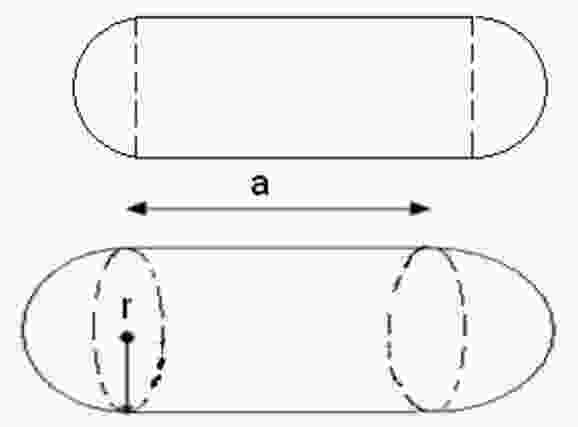### Capsule Volume, Surface Area and Circumference Calculation

The capsule is a shape consisting of a cylinder with hemispherical ends means a sphere has been cut in half through its center and combined at both the ends of a cylinder. The radius of sphere and cylinder is same, so we can say a cylinder with two hemispherical caps on either end. The formula used to calculate volume, surface area and circumference as follows:Volume = πr2((4/3)r + a)
Surface Area = 2πr(2r + a)
Circumference = 2πr
Where r is radius and a is side length.
 Capsule Volume Radius value (r) =m Side length (a) =m Volume of Capsule (V) =m3 Surface Area (S) =m2 Circumference (C) =m